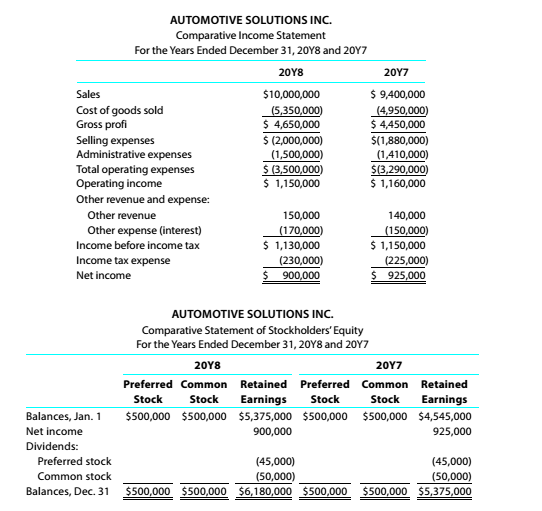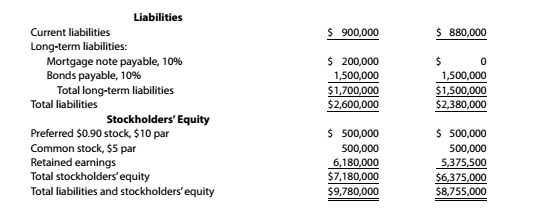Chapter 9, Problem 9.4.5P

Chapter
Section
Textbook Problem

Twenty metrics of liquidity, solvency, and profitabilityThe comparative financial statements of Automotive Solutions Inc. are as follows. The market price of Automotive Solutions Inc. common stock was $119.70 on December 31, 20Y8InstructionsDays’ sale in receivable To determine Concept Introduction: Accounts receivable turnover ratio: This is an efficiency ratio that indicates the conversion of the accounts receivable into cash. This ratio is calculated by dividing the Net credit Sales by the Average accounts receivable. The formula to calculate this ratio is as follows: Accounts receivable turnover ratio = Net credit SalesAverage accounts receivable Days Sales in receivable ratio: This is an efficiency ratio that indicates the period for which the credit sales remain as receivable. The ratio is calculated by dividing 365 days by the Accounts receivable turnover ratio. The formula to calculate this ratio is as follows: Days Sales in receivable ratio= 365Accounts receivable turnover ratio To Calculate: The Days Sales in Receivable. Explanation The Days Sales in Receivable is calculated as follows:  20Y8 Net Credit Sales (A)$ 10,000,000 Beginning Accounts Receivable (B) \$ 510,000

Still sussing out bartleby?

Check out a sample textbook solution.

See a sample solution

The Solution to Your Study Problems

Bartleby provides explanations to thousands of textbook problems written by our experts, many with advanced degrees!

Get Started